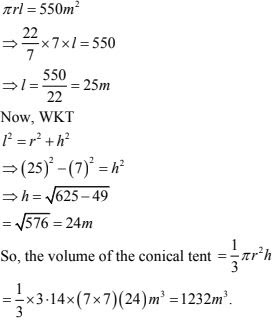# Chapter 20 Surface Area of Volume of a Right Circular Cone RD Sharma Solutions Exercise 20.2 Class 9 Maths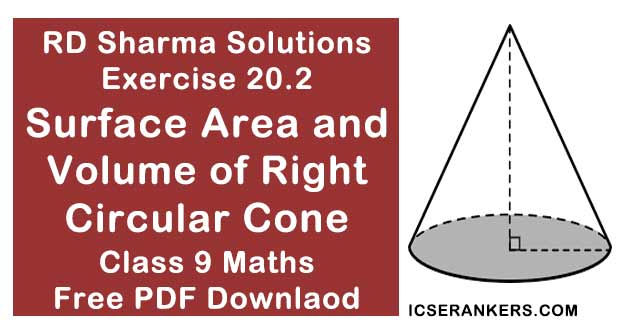Chapter Name RD Sharma Chapter 20 Surface Area of Volume of a Right Circular Cone Exercise 20.2 Book Name RD Sharma Mathematics for Class 10 Other Exercises Exercise 20.1 Related Study NCERT Solutions for Class 10 Maths

### Exercise 20.2 Solutions

1. Find the volume of a right circular cone with:
(i) radius 6 cm, height 7 cm.
(ii) radius 3.5 cm, height 12 cm
(iii) height 21 cm and slant height 28 cm.

Solution

(i) Given that ,
Radius of cone (r) = 6 m
Height of cone (h) = 7 cm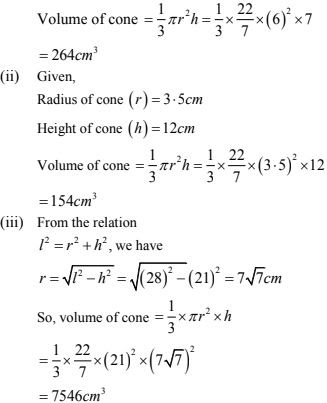2. Find the capacity in litres of a conical vessel with :
(i) radius 7 cm, slant height 25 cm
(ii) height 12 cm, slant height 13 cm.

Solution

(i) Radius of cone (r) = 7 cm
Slant height (l) of cone = 25 cm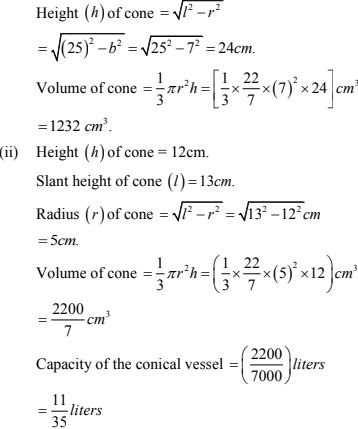3. Two cones have their heights in the ratio 1 : 3  and the radii of their bases in the ratio 3 : 1. Find the ratio of their volumes.
Solution
Given that, let height, h say
Height of 1st cone = h
Height of 2nd cone = 3h
Let the ratio of radii be r
∴ Radius of 1st cone = 3r
Radius of 2nd cone = r
∴ ratio of volume = V1 /V2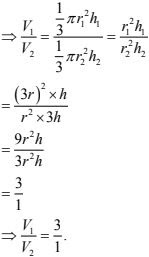4. The radius and the height of a right circular cone are in the ratio 5 : 12. If its volume is 314
cubic meter, find the slant height and the radius (Use it π = 3.14).
Solution
Let the ratio be x
Height 'h' = 12x
WKT,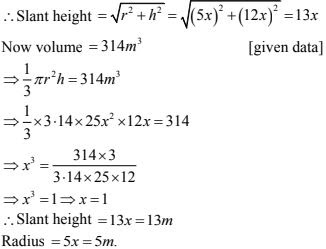5. The radius and height of a right circular cone are in the ratio 5 : 12 and its volume is 2512 cubic cm. Find the slant height and radius of the cone. (Use it π = 3.14).
Solution
Let the ratio be x
Height 'h' = 12x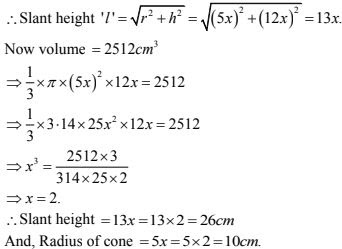6. The ratio of volumes of two cones is 4 : 5 and the ratio of the radii of their bases is 2:3. Find the ratio of their vertical heights.
Solution
Let ratio of radius be 'r'
Radius of 1st cone = 2r
Radius of 2nd cone = 3r
Similarly
Let volume ratio be 'v'
Volume of 1st cone → 4v
Similarly volume of 2nd cone → 5v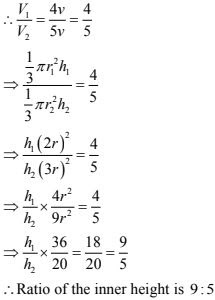7. A cylinder and a cone have equal radii of their bases and equal heights. Show that their volumes are in the ratio 3:1.
Solution
Given that,
A cylinder and a cone have equal radii of their equal bases and heights
Let height of cone = height of cylinder = h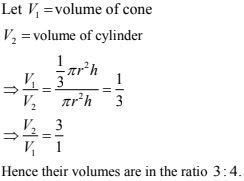8. If the radius of the base of a cone is halved, keeping the height same, what is the ratio of the volume of the reduced cone to that of the original cone?
Solution
Let radius of cone is r and height is h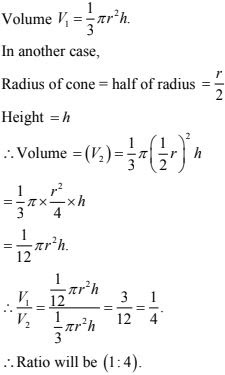9. A heap of wheat is in the form of a cone of diameter 9 m and height 3.5 m . Find its volume. How much canvas cloth is required to just cover the heap? (Use π = 3.14).
Solution
Diameter of heap d = 9 m
Radius = 9/2 m = 4.5 m.
Height (h) = 3.5 m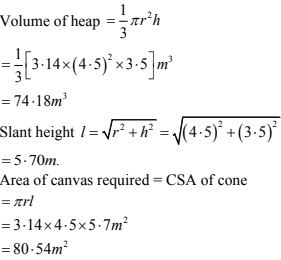10. A heap of wheat is in the form of a cone of diameter 9 m and height 3.5 m. Find its volume.
How much canvas cloth is required to just cover the heap? (Use π = 3.14).
Solution
Given diameter of cone 14 cm
∴ Radius of cone = 7 cm
Height of cone = 51 cm.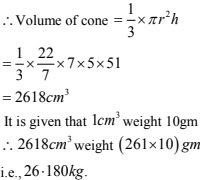11. A right angled triangle of which the sides containing he right angle are 6.3 cm and lo cm in length, is made to turn round on the longer side. Find the volume of the solid, thus generated. Also, find its curved surface area.
Solution
Given, radius of cone (r) = 6.3 cm
Height of cone (h) = 10 cm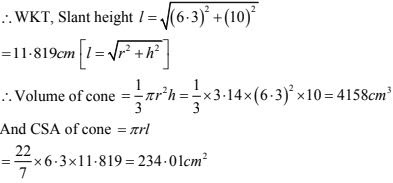12. Find the volume of the largest right circular cone that can be fitted in a cube whose edge is 14 cm.
Solution
For largest circular cone radius of the based of the cone = 1/2 edge of cube
= 1/2 × 14 = 7  cm
And height of the cone = 14 cm
Volume of cone = 1/3 × 3.14 ×7×7×14 = 718.666 cm3 .

13. The volume of a right circular cone is 9856 cm3 . If the diameter of the base is 28 cm, find :
(i) height of the cone
(ii) slant height of the cone
(iii) curved surface area of the cone.
Solution
(i) Radius of cone = 28/2 cm = 14 cm
Let height of cone is h
Volume of cone = 9856 cm3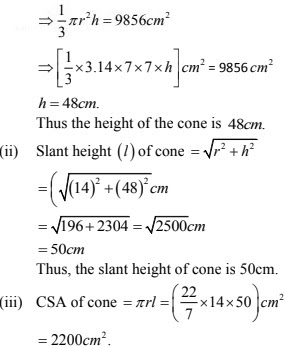14. A conical pit of top diameter 3.5 m is 12 m deep. What is its capacity in kilo litres ?
Solution
Radius (r) of pit = 3.5/2 m = 1.75 m.
Depth (h) of pit  = 12 m.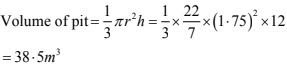∴ Capacity of the pit = (38.5 ×1) kilometers
= 38.5 kilo liters

15. Monica has a piece of Canvas whose area is 551 m2 . She uses it to have a conical tent made,
with a base radius of 7m. Assuming that all the stitching margins and wastage incurred while
cutting, amounts to approximately 1 m2 . Find the volume of the tent that can be made with it.
Solution
Given that,
Area of canvas = 551 m2  and area of the canvas lost in wastage is 1m2 .
∴area of canvas available for making the tent is (551 - 1)m2  = 552 m2 .
SA of tent = 550 m2  required base radius of conical tent = 7m.
CSA of tent = 550 m2